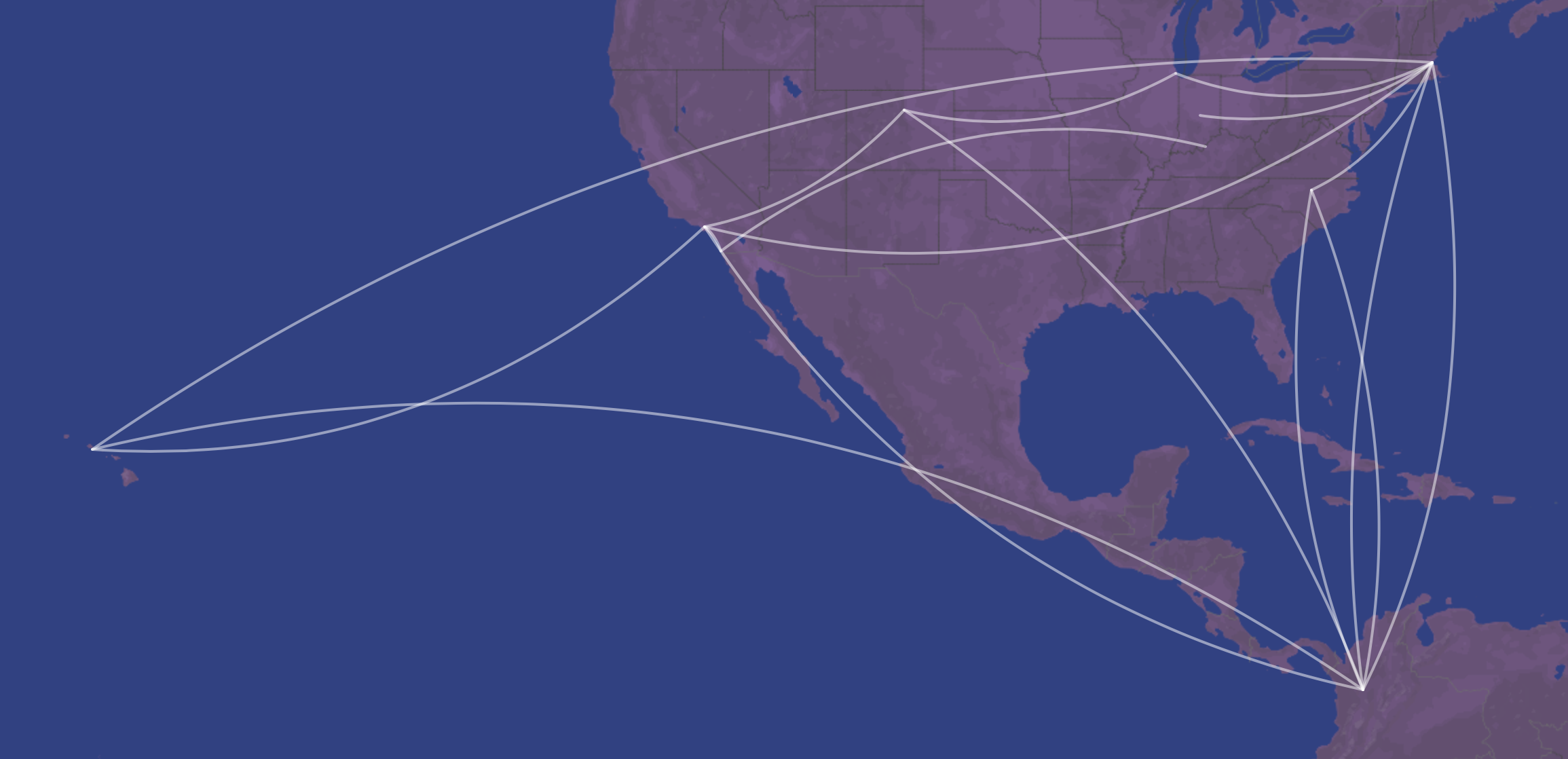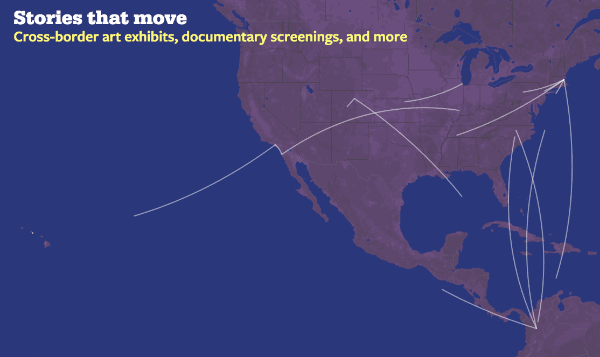# Creating consistently curved lines on Leaflet

## Finally, high school trigonometry comes in handyThe final product.

For my new personal website, I wanted to create a map with lines connecting various cities—easy enough with Leaflet. However, I couldn’t find an easy way to make those lines nicely curved. If the cities were far enough apart, there are great plugins for drawing “great circle” (or geodesic) lines, which take into account the curvature of the Earth, and thus appear as curved on a flat map. But the some of the lines I wanted to create were short enough that the great circle method wouldn’t consistently create curved lines.

Finally, I figured out a different way, which I must admit stretched the limits of my memory of high school trigonometry—so I’m not sure it’s the most efficient method. That said, it did accomplish exactly what I envisioned.

So, as a general example, say we have two points that we want to connect, which Leaflet is happy to do with a straight line (fig. 1)—but we want a curved line (fig. 2).

This can be achieved with Leaflet.curve, which can easily create Bézier curves—but we need to provide it with at least one control point (fig. 3). The question is thus how to find the coordinates of that point (fig. 4).

I eventually realized that the easiest way would be within the polar coordinate system. To do so, we first convert latitude and longitude into Cartesian coordinates. Longitude (east/west) is `x`, latitude (north/south) is `y` (fig. 5). We then offset both points so that one is at the origin, `(0,0)` (fig 6.). Finally, we convert the non-origin point to its polar coordinate `(r,θ)`. `r` is calculated by the Pythagorean theorem, and `θ` with the `atan2` function (fig. 7).

Now, we want to find a point on a line perpendicular to the midpoint of our original line. We want that line to be offset by some angle, which we’ll call `θoffset`. Thinking of this in terms of a right triangle (fig. 8), we know the value of `θ` (`θoffset`) and its adjacent side (`r/2`); we want to solve for the hypotenuse. Since `cosθ = adjacent / hypotenuse`, `hypotenuse = adjacent / cosθ`. We plug in those values and get new coordinates, `r2` and `θ2`.

We can then convert those back to Cartesian coordinates: `x = r*cosθ`, `y = r*sinθ` (fig 9). Then we move all the coordinates back to their original locations (fig 10)…

…and back to latitude and longitude, i.e. by switching `x` and `y` (fig. 11). From there, we just have to add `(latitude3,longitude3)` to the points that create the line (fig. 12). Leaflet.curve can then create a quadratic Bézier curve with the control point at `(latitude3,longitude3)` (fig. 13).

Finally, Leaflet.curve can also animate the line through the Web Animations API (on supported browsers). I came up with this:See the full animation on supported browsers here.

The Javascript code for all of this is below and on GitHub: